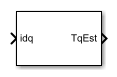# PMSM Torque Estimator

Estimate permanent magnet synchronous machine torque

• Library:
• Simscape / Electrical / Control / PMSM Control

•## Description

The PMSM Torque Estimator block implements a torque estimator for permanent magnet synchronous machines (PMSM).

Use this block to estimate the mechanical torque of a motor when it is not directly measurable. The block estimates torque using known machine parameters and the measured phase current vector in the dq0 reference frame.

Use the Park Transform block to convert the measured phase current vector in the abc reference frame to the dq0 reference frame.

### Equations

The block estimates the mechanical torque Te of the PMSM using the torque equation in the d-q rotor reference frame:

`${T}_{e}=\frac{3p}{2}\left({\psi }_{m}{i}_{q}+\left({L}_{d}-{L}_{q}\right){i}_{d}{i}_{q}\right),$`

where

• p is the number of pole pairs of the PMSM.

• ψm is the flux linkage of the permanent magnet.

• Ld and Lq are the d- and q-axis inductances of the PMSM.

• id and iq are the d- and q-axis currents of the PMSM.

In practice, the machine parameters are not constants and depend on some physical phenomena. You can choose to define these parameters simply as constants or, more realistically, as functions of currents by using lookup tables.

### Assumptions

The machine parameters are known.

## Ports

### Input

expand all

Stator direct and quadrature currents of the PMSM, in A.

Data Types: `single` | `double`

### Output

expand all

Estimated mechanical torque value of the PMSM, in N*m.

Data Types: `single` | `double`

## Parameters

expand all

Specify the type of machine parameters, which can be in the form of constant values or tabulated data.

Number of permanent magnet pole pairs on the rotor.

Direct-axis current vector used in the lookup tables for parameters determination.

Quadrature-axis current vector used in the lookup tables for parameters determination.

Ld matrix used as lookup table data.

Lq matrix used as lookup table data.

Permanent magnet flux linkage matrix used in the lookup table.

Direct-axis inductance.

Sample time for the block (-1 for inherited). If you use this block inside a triggered subsystem, set the sample time to `-1`. If you use this block in a continuous variable-step model, set the sample time explicitly.

## Version History

Introduced in R2017b